SLOS890C October   2015  – December 2019

PRODUCTION DATA.

1. Features
2. Applications
3. Description
1.     Device Images
4. Revision History
5. Device Comparison Table
6. Pin Configuration and Functions
7. Specifications
8. Detailed Description
1. 8.1 Overview
2. 8.2 Functional Block Diagram
3. 8.3 Feature Description
4. 8.4 Device Functional Modes
9. Application and Implementation
1. 9.1 Application Information
2. 9.2 Typical Application
10. 10Power Supply Recommendations
11. 11Layout
12. 12Device and Documentation Support
13. 13Mechanical, Packaging, and Orderable Information

• DCK|5
• DBV|5
• DCK|5

### 7.7 Typical Characteristics

at TA = 25°C, VS = 5 V, RPULLUP = 5.1 kΩ, and input overdrive = 100 mV (unless otherwise noted)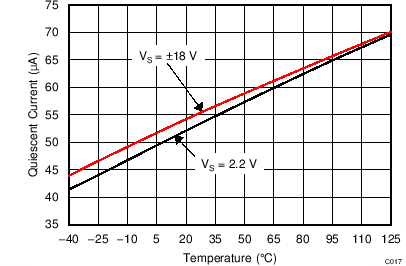Figure 1. Quiescent Current vs TemperatureFigure 3. Input Offset Current vs Temperature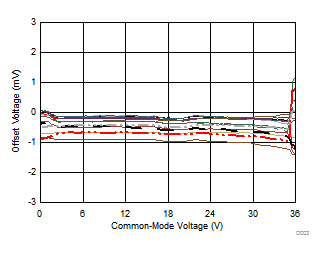VS = ±18 V 14 typical units shown
Figure 5. Offset Voltage vs Common-Mode Voltage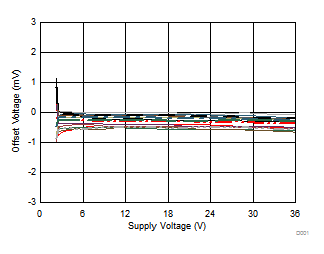16 typical units shown
Figure 7. Offset Voltage vs Supply Voltage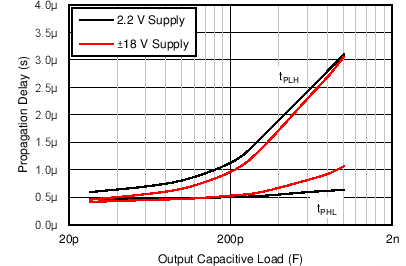Figure 9. Propagation Delay vs Capacitive Load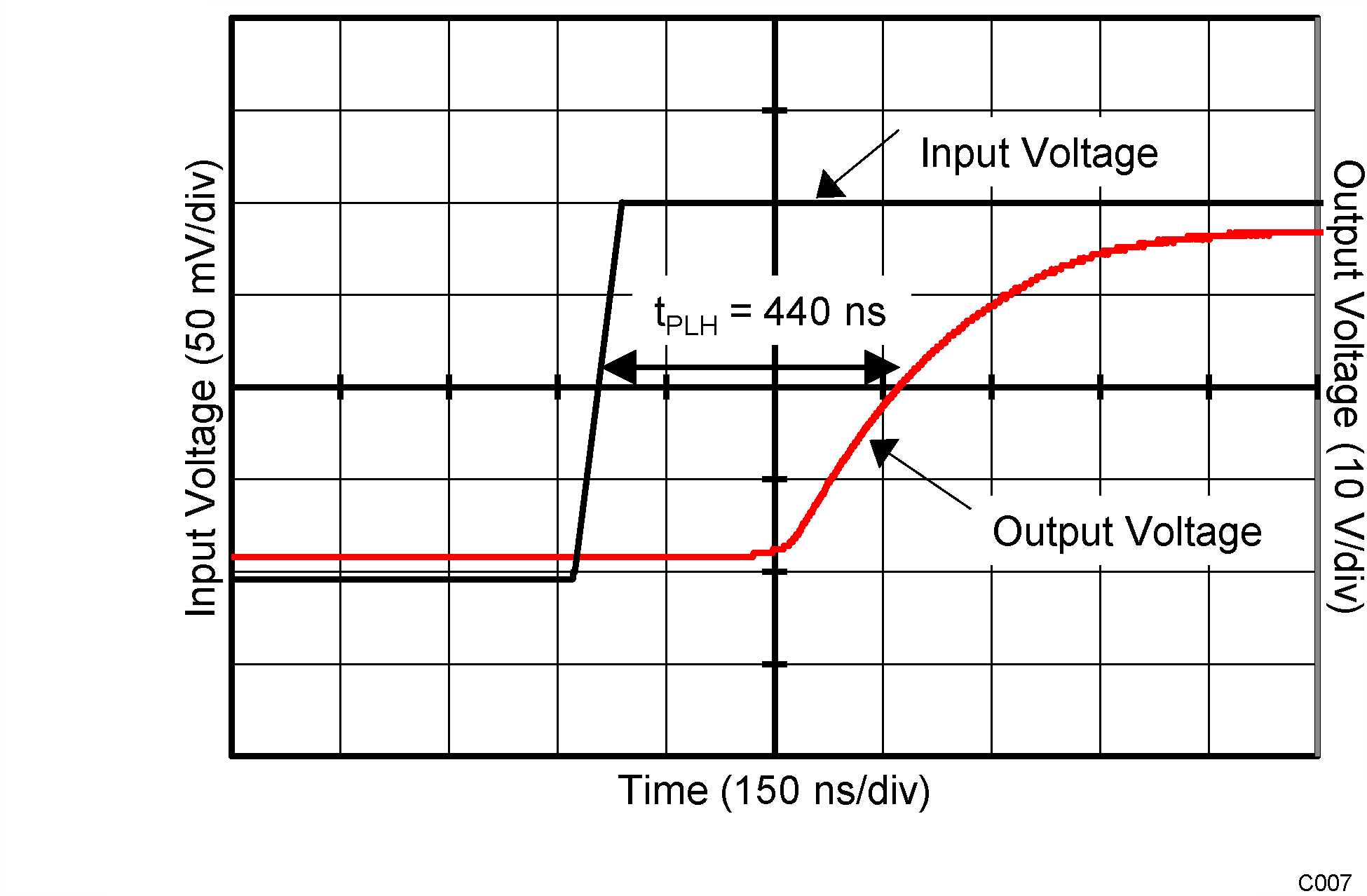VS = 36 V Overdrive = 100 mV
Figure 11. Propagation Delay (TpLH)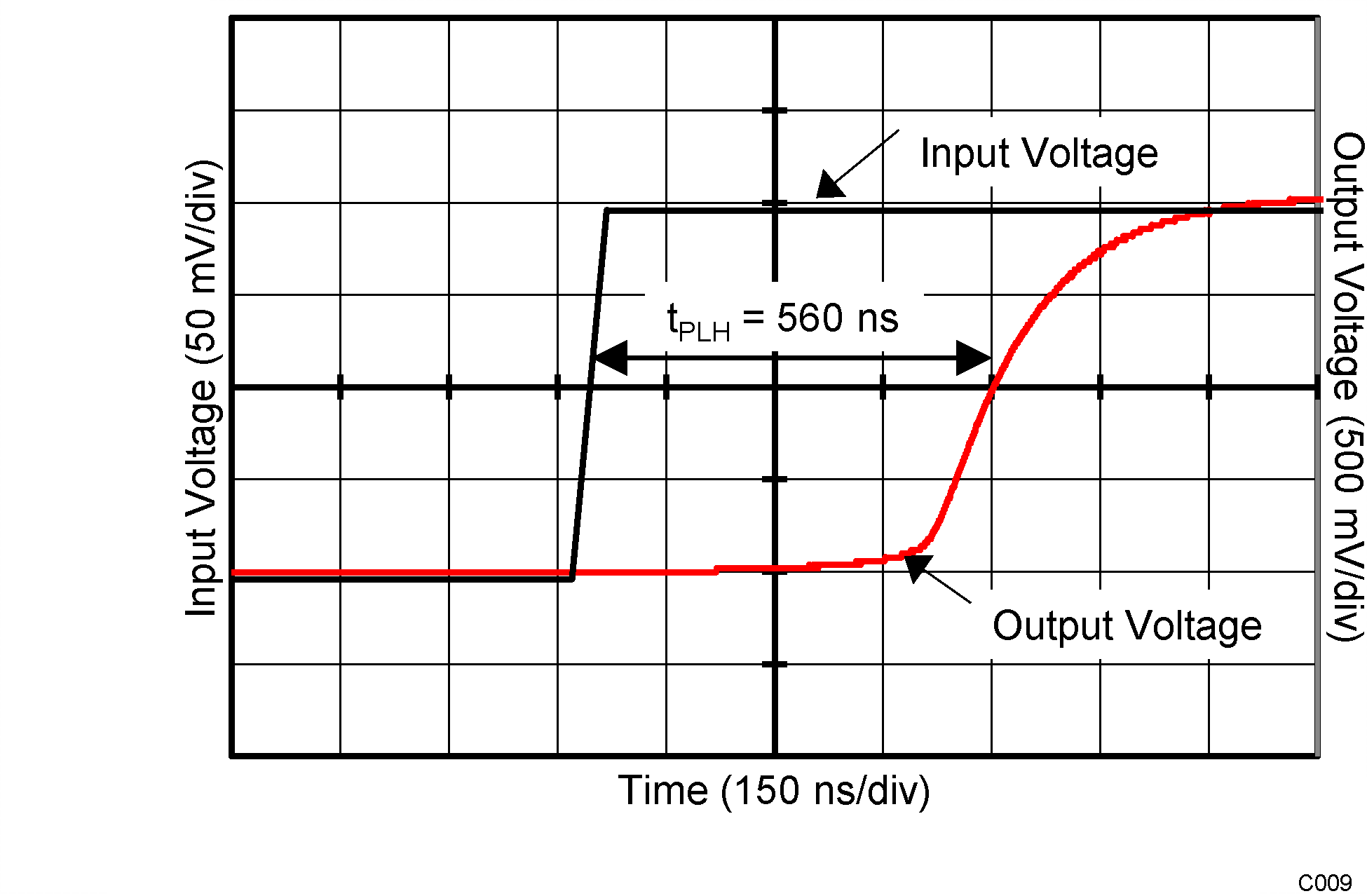VS = 2.2 V Overdrive = 100 mV
Figure 13. Propagation Delay (TpLH)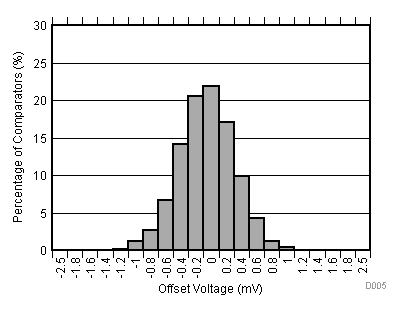VS = ±18 V Distribution taken from 2524 comparators
Figure 15. Offset Voltage Production Distribution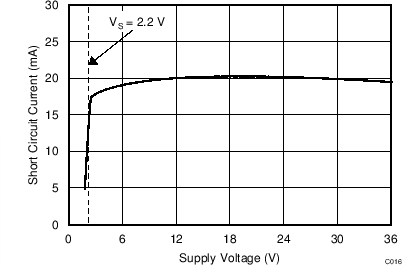Sink current
Figure 17. Short-Circuit Current vs Supply Voltage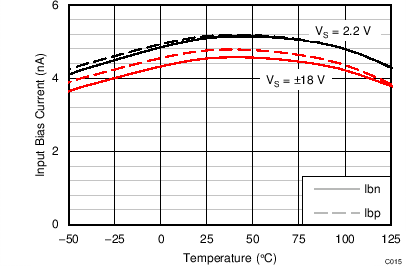Figure 2. Input Bias Current vs Temperature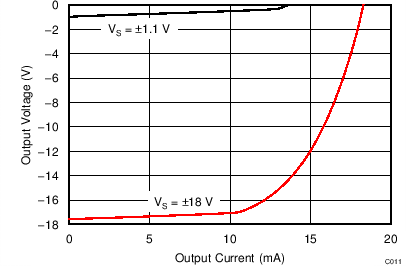Figure 4. Output Voltage vs Output Current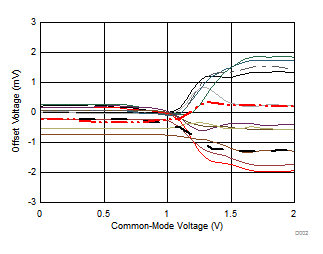VS = 2.2 V 13 typical units shown
Figure 6. Offset Voltage vs Common-Mode Voltage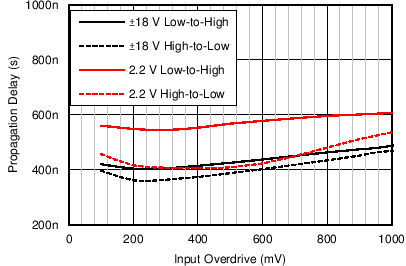Figure 8. Propagation Delay vs Input Overdrive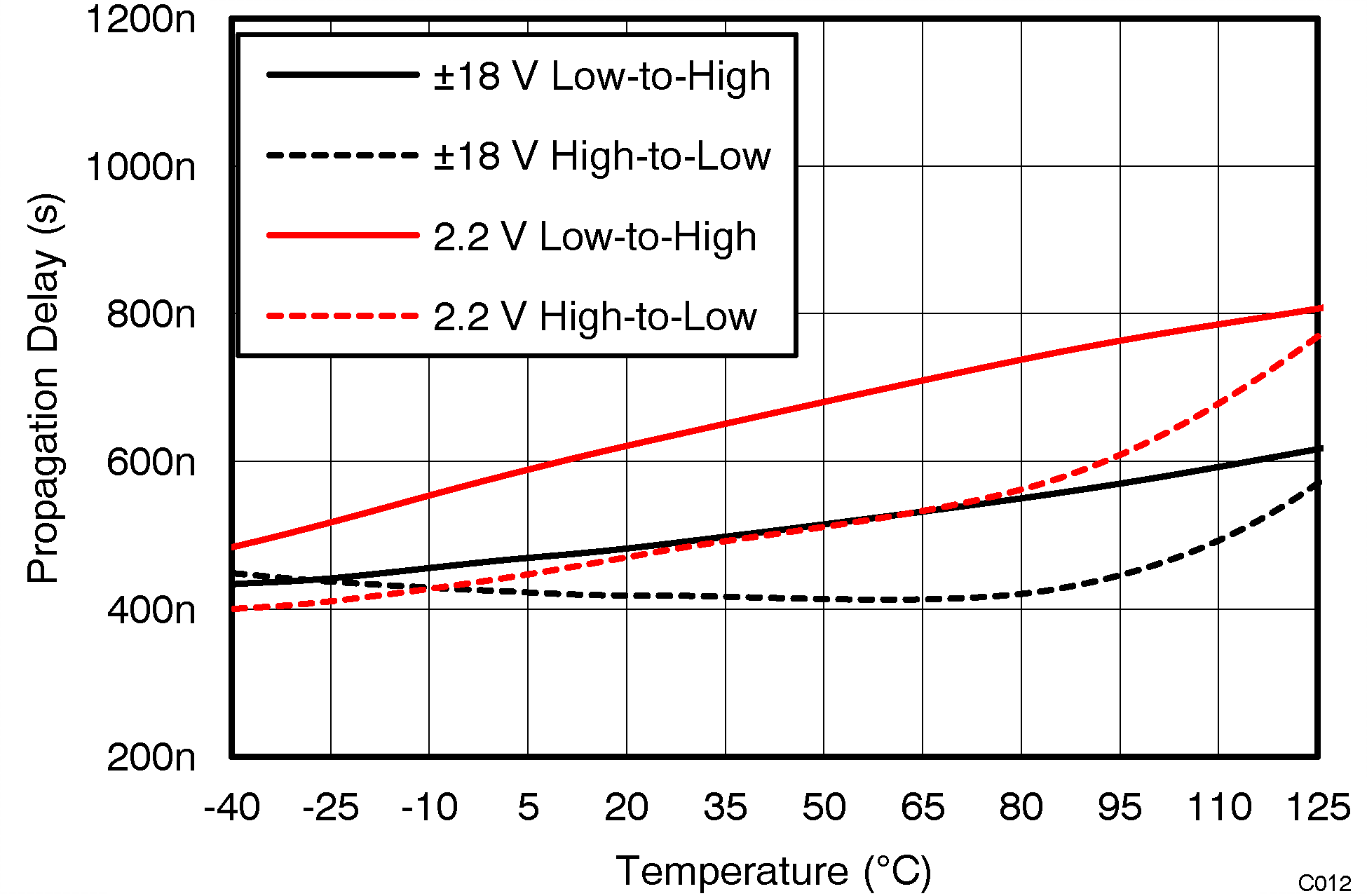VOD = 100 mV
Figure 10. Propagation Delay vs Temperature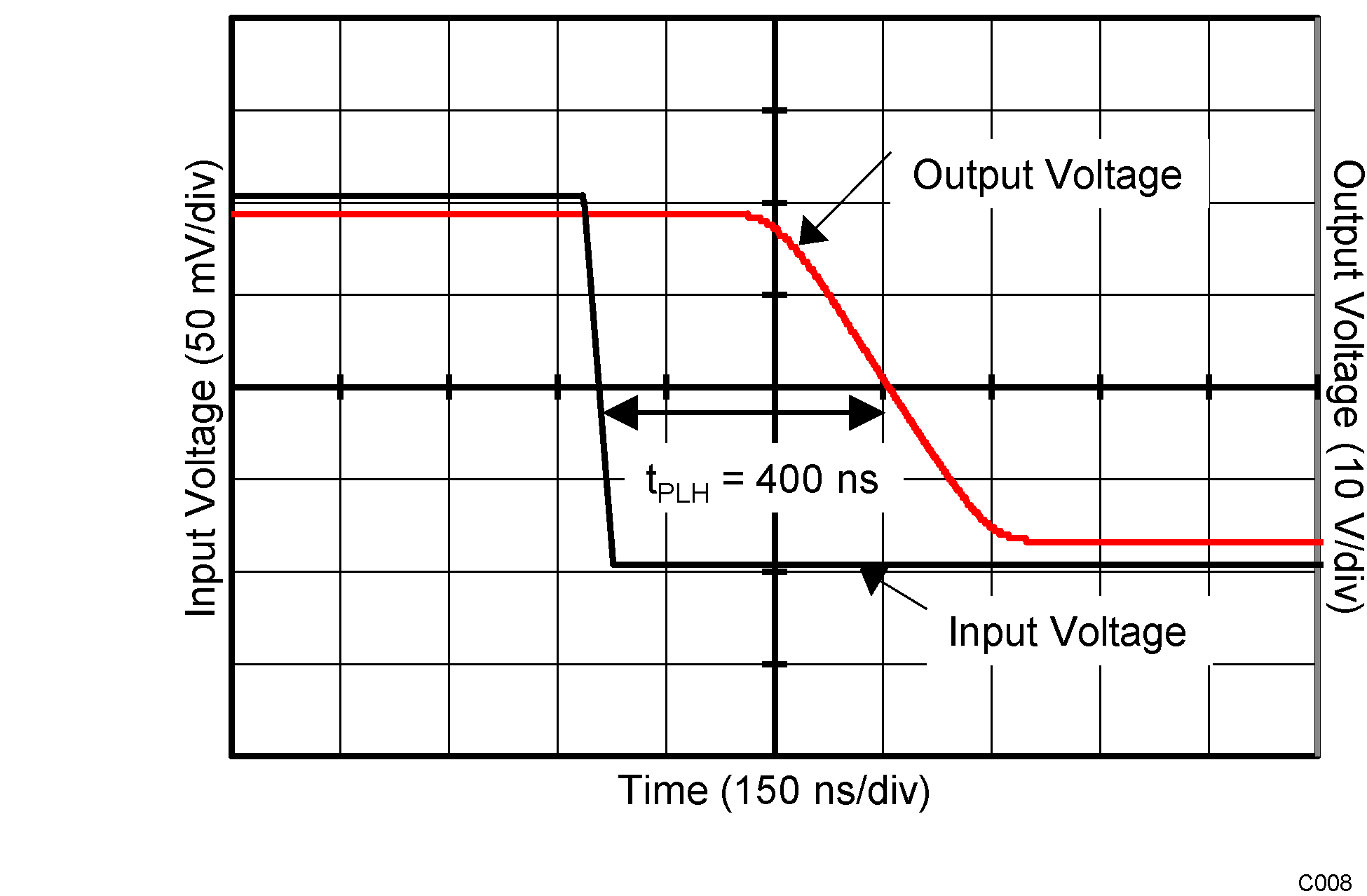VS = 36 V Overdrive = 100 mV
Figure 12. Propagation Delay (TpHL)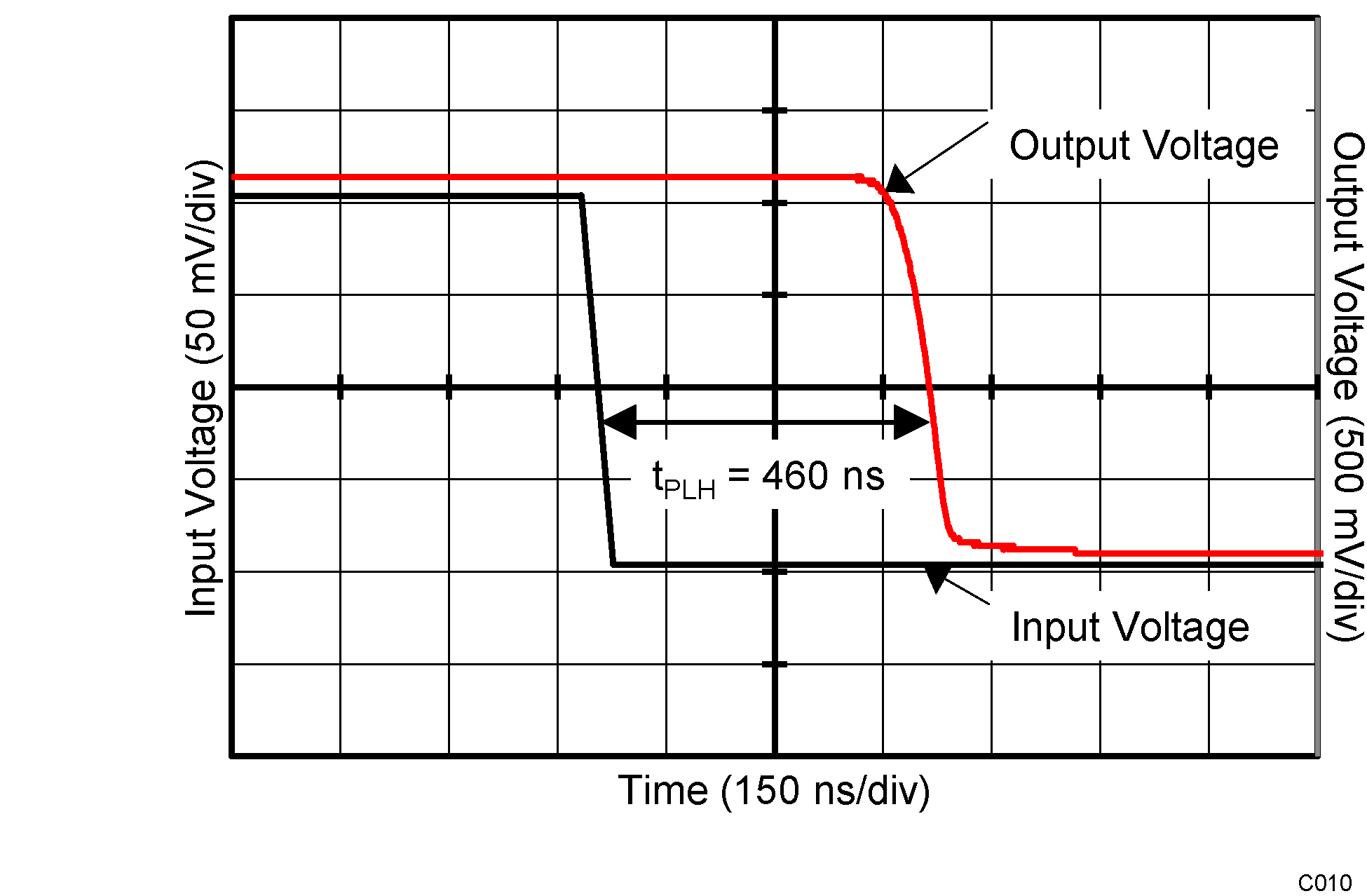VS = 2.2 V Overdrive = 100 mV
Figure 14. Propagation Delay (TpHL)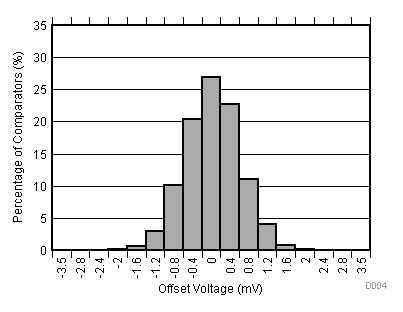VS = 2.2 V Distribution taken from 2524 comparators
Figure 16. Offset Voltage Production Distribution